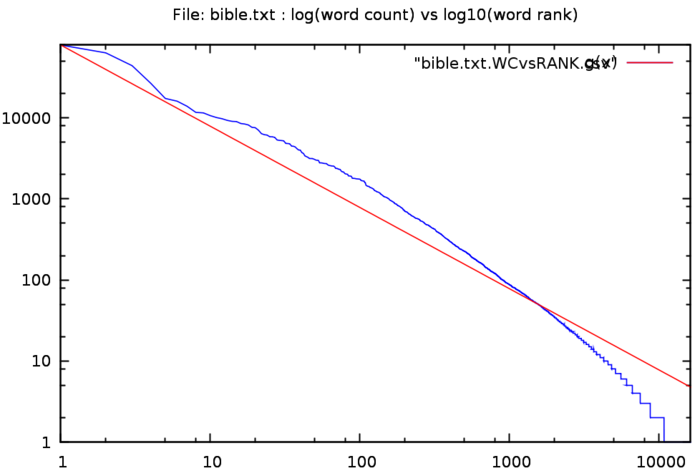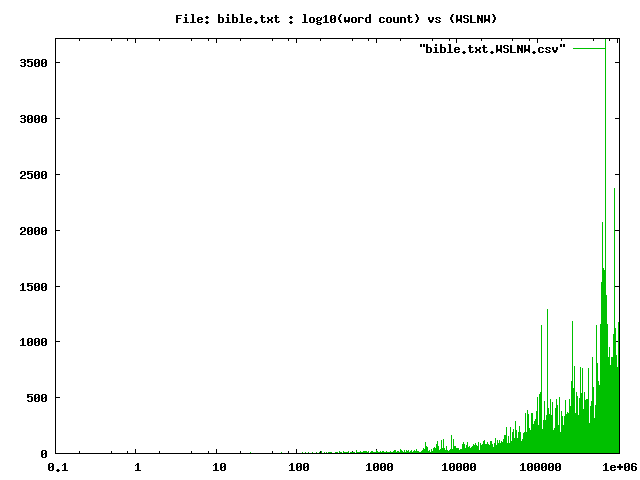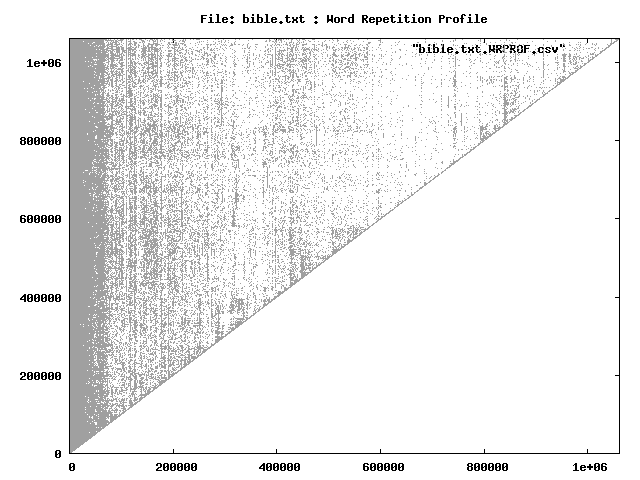Statistics on the text file bible.txt, using the command:zwc -s -d -l -o outfiles -f bible.txt The total word count is 1061230 The unique words number 16364 The ratio unique/total is 1.5420 % The highest ranking 54 words make up 50.035 % of the total, OR 0.330 % of the unique words make up 50.035 % of the total volume The number of words used only once 5510 The ratio (words used only once)/total is 0.5192 % The average of the ratios between frequencies is 1.0008